# KLM triangle

Find the length of the sides of the triangle KLM if m = 5cm height to m = 4.5 cm and size MKL angle is 70 degrees.

k =  5.6173 cm

### Step-by-step explanation:

Try calculation via our triangle calculator.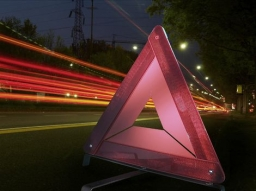Did you find an error or inaccuracy? Feel free to write us. Thank you!Tips to related online calculators
Pythagorean theorem is the base for the right triangle calculator.

#### You need to know the following knowledge to solve this word math problem:

We encourage you to watch this tutorial video on this math problem:

## Related math problems and questions:

• Right angled triangle 3Side b = 1.5, hypotenuse angle A = 70 degrees, Angle B = 20 degrees. Find its unknown sides length.
• Right triangleIt is given a right triangle angle alpha of 90 degrees beta angle of 55 degrees c = 10 cm use Pythagorean theorem to calculate sides a and b
• Right triangle trigonometricsCalculate the size of the remaining sides and angles of a right triangle ABC if it is given: b = 10 cm; c = 20 cm; angle alpha = 60° and the angle beta = 30° (use the Pythagorean theorem and functions sine, cosine, tangent, cotangent)
• Acute triangle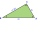In the acute triangle KLM, V is the intersection of its heights and X is the heel of height to the side KL. The axis of the angle XVL is parallel to the side LM and the angle MKL is 70°. What size are the KLM and KML angles?
• Right triangleA right triangle ABC is given, c is a hypotenuse. Find the length of the sides a, b, the angle beta if c = 5 and angle alfa = A = 35 degrees.
• 4side pyramidCalculate the volume and surface of 4 sides regular pyramid whose base edge is 4 cm long. The angle from the plane of the sidewall and base plane is 60 degrees.
• Isosceles triangle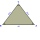Calculate the size of the interior angles and the length of the base of the isosceles triangle if the length of the arm is 17 cm and the height to the base is 12 cm.
• Rotary coneThe volume of the rotation of the cone is 472 cm3, and the angle between the side of the cone and the base angle is 70°. Calculate the lateral surface area of this cone.
• RightDetermine angles of the right triangle with the hypotenuse c and legs a, b, if: 3a +4b = 4.9c
• Cone sideCalculate the volume and area of the cone whose height is 10 cm and the axial section of the cone has an angle of 30 degrees between height and the cone side.
• Perimeter of triangleIn the triangle, ABC angle A is 60° angle B is 90°, and side size c is 15 cm. Calculate the triangle circumference.
• Rectangular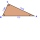Rectangular triangle KLM with right angle at vertex L, angle beta at vertex K and angle alpha at vertex M. Angle at vertex M = 65°, side l = 17.5 cm. Use Pythagorean theorems and trigonometric functions to calculate the lengths of all sides and the angle
• Euclid 5Calculate the length of remain sides of a right triangle ABC if a = 7 cm and height vc = 5 cm.
• Triangle and its heightsCalculate the length of the sides of the triangle ABC, if va=5 cm, vb=7 cm and side b is 5 cm shorter than side a.
• Right angleIn a right triangle ABC with a right angle at the apex C, we know the side length AB = 24 cm and the angle at the vertex B = 71°. Calculate the length of the legs of the triangle.
• A rhombusA rhombus has sides of length 10 cm, and the angle between two adjacent sides is 76 degrees. Find the length of the longer diagonal of the rhombus.
• Angles and sides of the triangle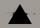Triangle ABC has a circumference of 26 cm. Lengths of the sides are as follows: a = 11.2 cm; b = 6.5 cm. Arrange the interior angles in order of their size. ...